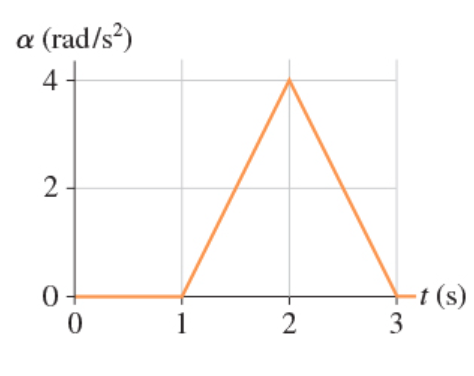# Problem: A wheel initially rotating at 57 rpm experiences the angular acceleration shown in (Figure 1) .What is the wheel's angular velocity, in rpm, at t = 3.0 s?

###### FREE Expert Solution

The area under the α - t graph gives ω.

Initial angular velocity, ωi = 57 rpmWhat is the wheel's angular velocity, in rpm, at t = 3.0 s?